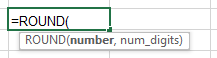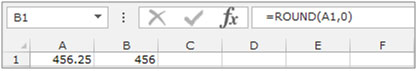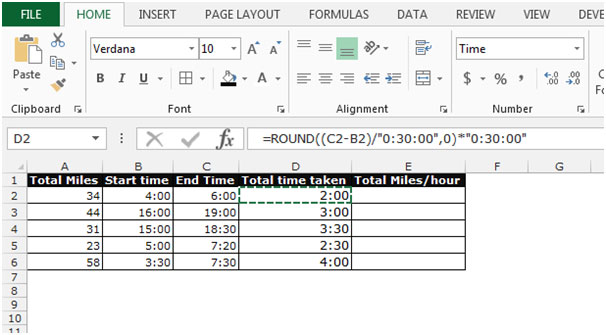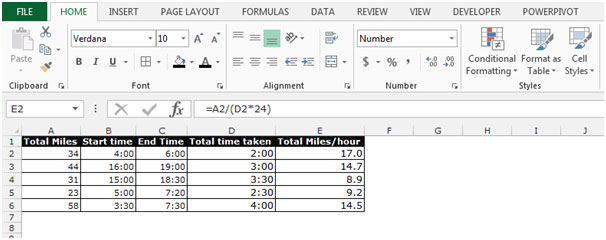# Calculate Average Speed in Microsoft Excel

In this article, we will learn how to calculate average speed in Microsoft Excel. To calculate the average speed, we have to divide the total distance by total time. We will use the “Round” function to return the total taken hours.

ROUND: - This function is used for rounding a number to a specified number.For Example: Cell A1 is contains the number 456.25 To round the number, follow below given steps:-

• Write the formula in cell B1.
• =ROUND (A1, 0), and the function will return 456Let’s take an example to understand how to calculate the average speed:-

Column A contains total miles, column B contains Start time, and column C contains End time. Column D will return the total time in hours. Column E will return the total miles per hour.To return the total taken hours, follow the below given steps:-

• Write the formula in cell D2.
• =ROUND((C2-B2)/"0:30:00",0)*"0:30:00"
• Press Enter on your keyboard.
• The function will round the times down to the nearest 30 seconds.
• Copy the same formula by pressing the key Ctrl+C and paste in the range D3:D6 by pressing the key Ctrl+V on your keyboard.The formula to calculate average speed, follow below steps:-

• Select the cell E2.
• Write the formula =A2/(D2*24)
• Press Enter on your keyboard.
• The function will return the Miles per hour.
• Copy the same formula by pressing the key Ctrl+C and paste in the range D3:D6 by pressing the key Ctrl+V on your keyboard.This is the way by which average speed calculator will work in Microsoft Excel.We would love to hear from you, do let us know how we can improve, complement or innovate our work and make it better for you. Write us at info@exceltip.com

1.How do we deal with minutes?

2.Your formula actually rounds to nearest half hour (30 minutes) not 30 seconds as it shows...

3.Rustom HAVEWALLA

Very useful.
Came to know for the first time that the hours had to be divided by 24.

Terms and Conditions of use

The applications/code on this site are distributed as is and without warranties or liability. In no event shall the owner of the copyrights, or the authors of the applications/code be liable for any loss of profit, any problems or any damage resulting from the use or evaluation of the applications/code.